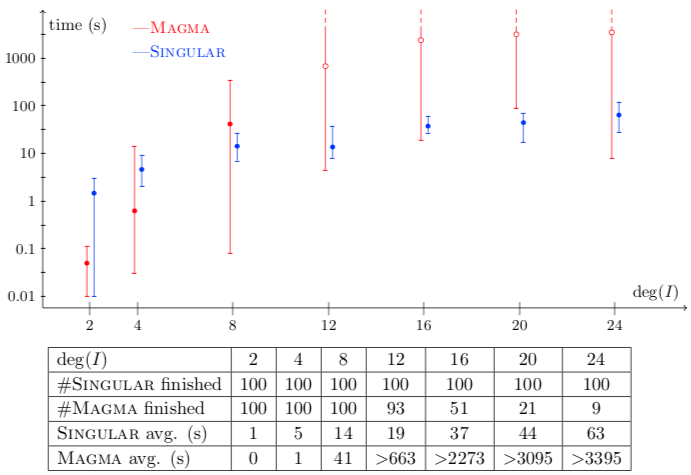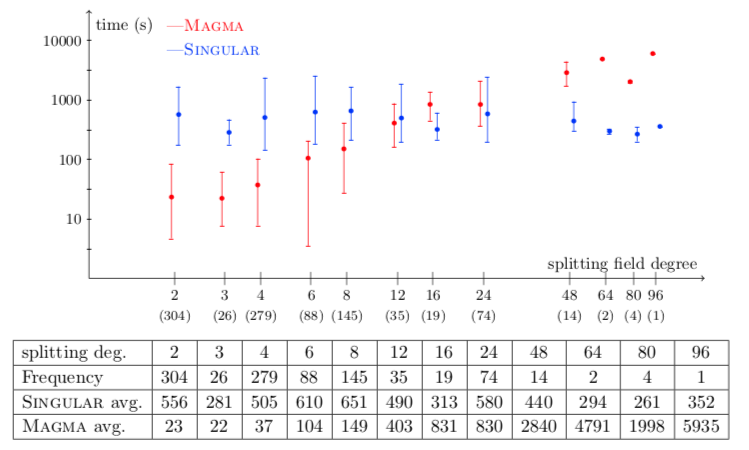# Examples¶

All examples are for $$K=\mathbb Q$$ equipped with the 2-adic valuation.

## Random lexicographical Groebner bases of ideals in shape position¶

A random lexicographical Groebner basis $$G$$ of an ideal $$I\subseteq \mathbb Q[x_1,\ldots,x_n]$$ in shape position is of the form

$\{ f_n(x_n), x_{n-1} - f_{n-1}(x_n), \ldots, x_1-f_1(x_n) \},$

where

• $$f_n,f_{n-1},\ldots,f_1$$ are univariate polynomials of degrees $$d,d-1,\ldots,d-1$$ respectively,

• all coefficients are of the form $$2^\lambda \cdot (2k+1)$$ for some random valuation $$0\leq\lambda < 100$$ and some random $$0\leq k < 5000$$.

In our paper, we have constructed 100 random lexicographical Groebner bases for $$d=2,4,8,12,16,20,24$$. You can load all examples into Singular by running the script as follows:

                     SINGULAR                                /  Development
A Computer Algebra System for Polynomial Computations       /   version 4.1.1
0<
by: W. Decker, G.-M. Greuel, G. Pfister, H. Schoenemann     \   Feb 2018
FB Mathematik der Universitaet, D-67653 Kaiserslautern       \
> <"lexicographicalGroebnerBases.sing".


Differentiated by degree, the timings are:## 27 distinct tropical lines on a random honeycomb cubic¶

In [PV19], it is shown that a general tropical cubic surface $$\text{Trop}(f)$$ in $$\mathbb T\mathbb P^3$$ in honeycomb form contains 27 distinct tropical lines, which must then be tropicalizations of the 27 lines on any algebraic cubic $$\text{V}(f)$$ in $$\mathbb P^3$$.

In our paper, we have constructed 1000 random cubic polynomials, whose coefficients are pure powers of 2, and computed the lines on the tropical cubic surfaces as points in $$\text{Grass}(2,4)$$ in the affine chart where Pluecker coordinate $$p_{34}$$ is 1.

You can load all examples into Singular by running the script as follows:

                     SINGULAR                                /  Development
A Computer Algebra System for Polynomial Computations       /   version 4.1.1
0<
by: W. Decker, G.-M. Greuel, G. Pfister, H. Schoenemann     \   Feb 2018
FB Mathematik der Universitaet, D-67653 Kaiserslautern       \
> <"timingExamplesCubics.sing".


Differentiated by splitting field, the timings are: